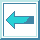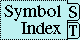Special Operator THE

Syntax:

the value-type form => result*

Arguments and Values:

value-type---a type specifier; not evaluated.

form---a form; evaluated.

results---the values resulting from the evaluation of form. These values must conform to the type supplied by value-type; see below.

Description:

the specifies that the values[1a] returned by form are of the types specified by value-type. The consequences are undefined if any result is not of the declared type.

It is permissible for form to yield a different number of values than are specified by value-type, provided that the values for which types are declared are indeed of those types. Missing values are treated as nil for the purposes of checking their types.

Regardless of number of values declared by value-type, the number of values returned by the the special form is the same as the number of values returned by form.

Examples:

(the symbol (car (list (gensym)))) =>  #:G9876
(the fixnum (+ 5 7)) =>  12
(the (values) (truncate 3.2 2)) =>  1, 1.2
(the integer (truncate 3.2 2)) =>  1, 1.2
(the (values integer) (truncate 3.2 2)) =>  1, 1.2
(the (values integer float) (truncate 3.2 2))   =>  1, 1.2
(the (values integer float symbol) (truncate 3.2 2)) =>  1, 1.2
(the (values integer float symbol t null list)
(truncate 3.2 2)) =>  1, 1.2
(let ((i 100))
(declare (fixnum i))
(the fixnum (1+ i))) =>  101
(let* ((x (list 'a 'b 'c))
(y 5))
(setf (the fixnum (car x)) y)
x) =>  (5 B C)

Affected By: None.

Exceptional Situations:

The consequences are undefined if the values yielded by the form are not of the type specified by value-type.

values

Notes:

The values type specifier can be used to indicate the types of multiple values:

(the (values integer integer) (floor x y))
(the (values string t)
(gethash the-key the-string-table))

setf can be used with the type declarations. In this case the declaration is transferred to the form that specifies the new value. The resulting setf form is then analyzed.

The following X3J13 cleanup issues, not part of the specification, apply to this section: